# NCERT Solutions for Class 9 Maths Chapter 7 - Triangles

Share

The NCERT Solutions For Class 9 Maths Chapter 7 - Triangles presented here are one of the most important resources for exam preparation. The Chapter comprises of important topics such as Congruence Of Triangles, Some Properties Of A Triangle and Inequalities In A Triangle. All these topics have been dealt with properly and explanations for them have been crafted using examples and illustrations. So, students who want to prepare the Chapter Triangle in a thorough manner can refer to our Solutions for the Chapter.

The Solutions have been developed by the experts of the Subject and they follow strictly the updated syllabus of the CBSE. Using these NCERT Solutions, students can find simple to understand explanations for important topics in the Chapter. All the Solutions are provided step-by-step and can be browsed easily without having to spend much time. So, just refer to these NCERT Solutions and give yourself the best chance to prepare for the exam.

## NCERT Solutions for Class 9 Maths Chapter 7 - Triangles

Exercise 7.1
• Exercise 7.1
• Exercise 7.2
• Exercise 7.3
• Exercise 7.4
• Exercise 7.5
Class 9th|NCERT - MathematicsChapter 7 - Triangles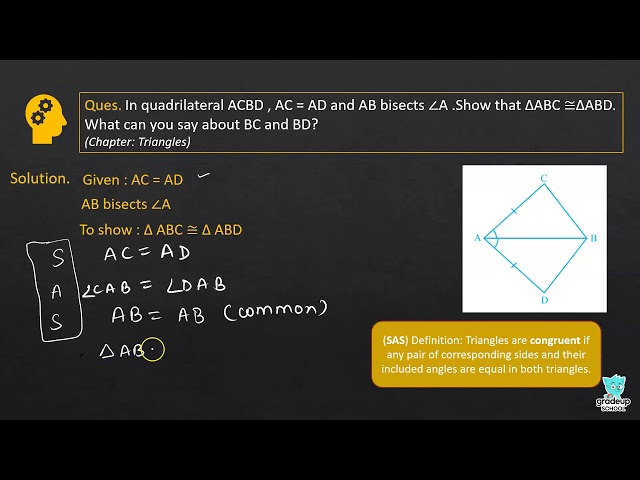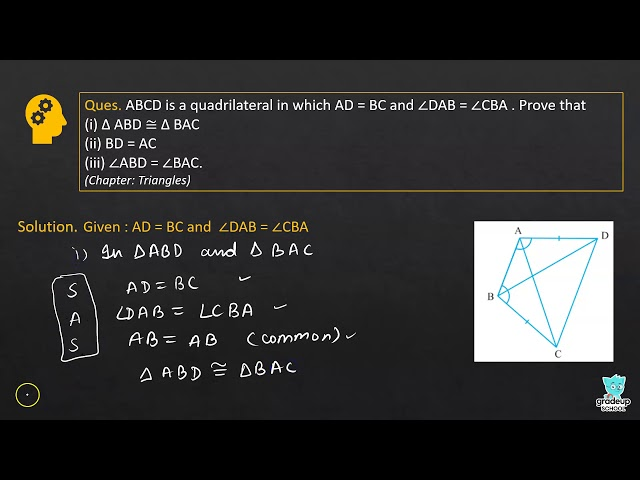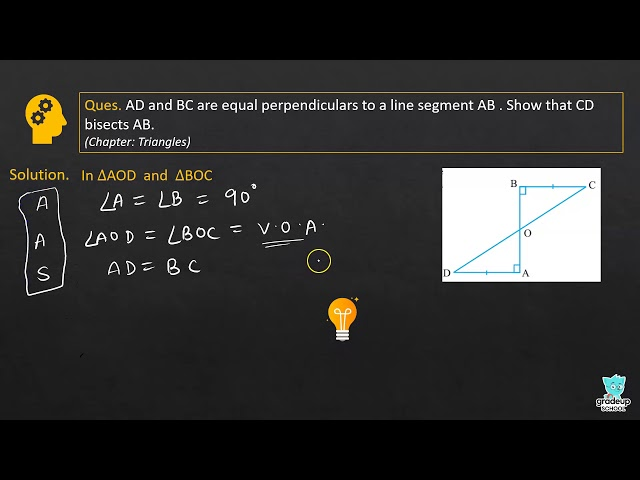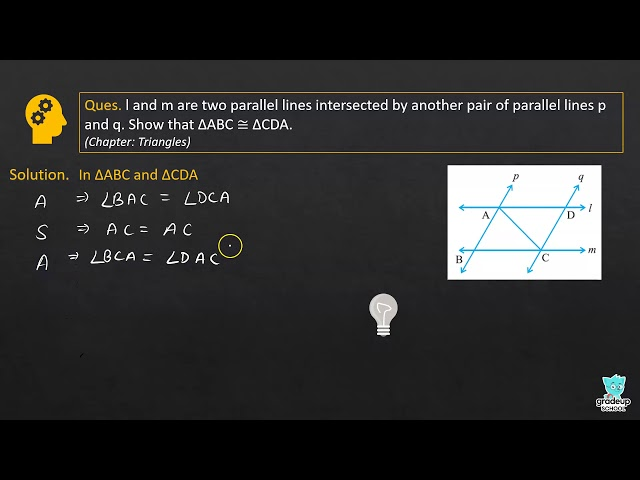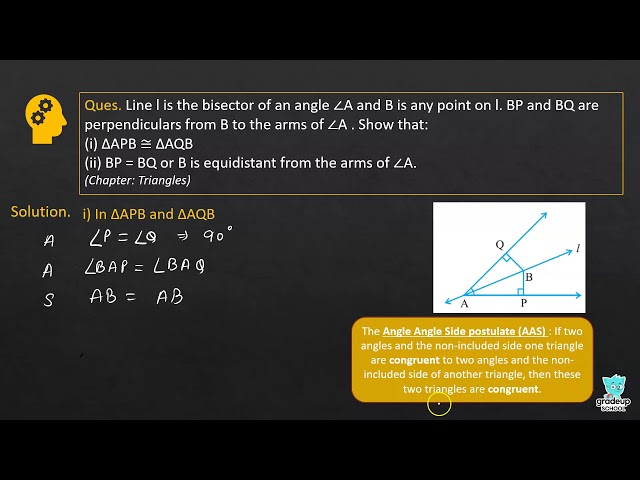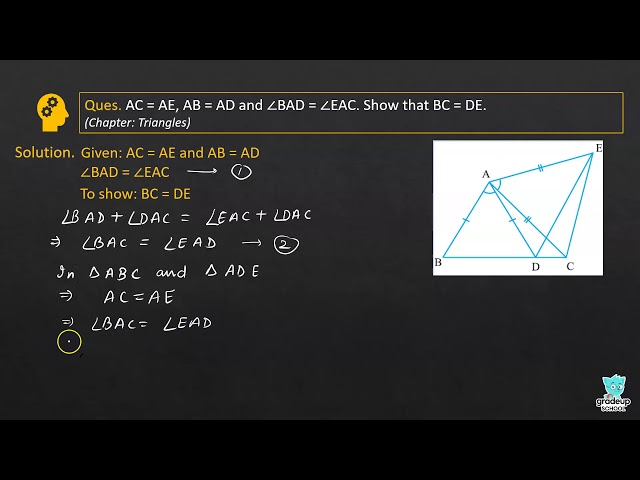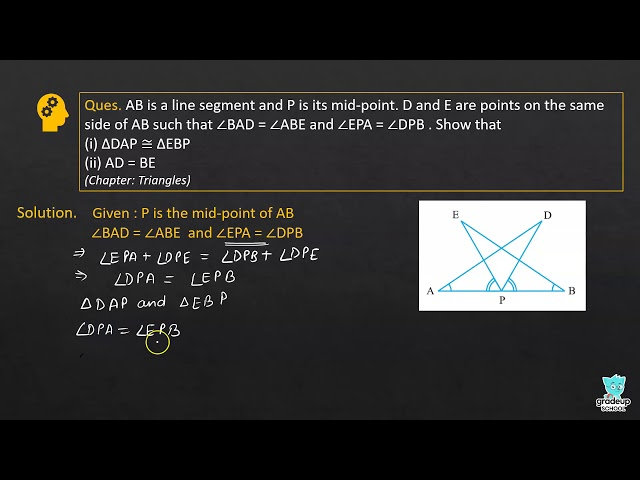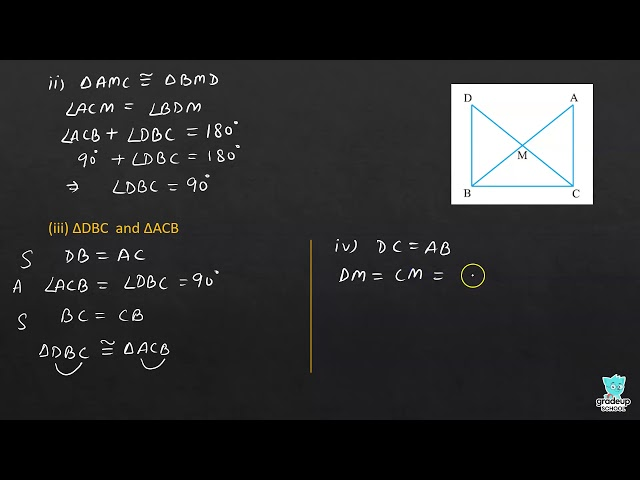NCERT - MathematicsClass 9th , NCERT
 Chapter 1 - Number System Chapter 2 - Polynomials Chapter 3 - Coordinate Geometry Chapter 4 - Linear Equations in two Variables Chapter 5 - Introduction to Euclid's Geometry Chapter 6 - Lines and Angles Chapter 7 - Triangles Chapter 8 - Quadrilaterals Chapter 9 - Areas of Parallelograms and Triangles Chapter 10 - Circles Chapter 11 - Constructions Chapter 12 - Heron's Formula Chapter 13 - Surface Areas and Volumes Chapter 14 - Statistics Chapter 15 - Probability